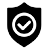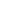#### WhatsApp Quick Chat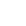#### Get Instant Call Back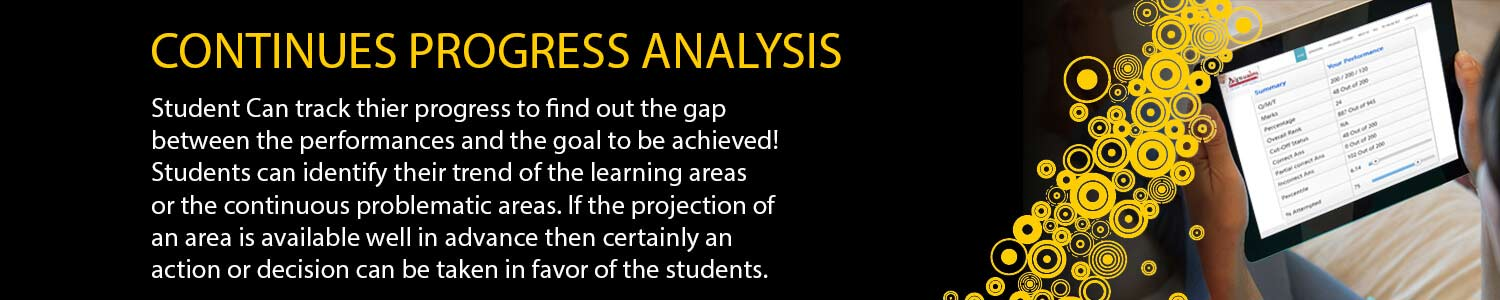## IIT JAM MATH

#### Offers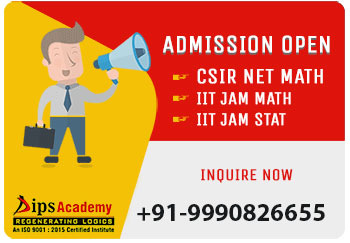##### News & Events# IIT JAM Maths Online Test Series Schedule

### IIT-JAM MATH TEST SERIES SCHEDULE 2022 (ONLINE)

TEST TYPE Modules DATE Syllabus
MWT-01 Real Analysis:1 3-Jan-22 Sequences and Series of Real Number: Convergence of sequences, bounded and monotone sequences, Cauchy sequences, Bolzano-Weierstrass theorem, absolute convergence, tests of convergence for series-comparison test, ratio test, root test; Power series (of one real variabel), radius and interval of convergence, term-wise differentiation and integration of power series.
MWT-02 Group Theory 6-Jan-22 Groups: Cyclic groups, abelian groups, non-abelian groups, permutation gropus, normal subgroups, quotient groups, Lagrange's theorem for finite groups, group homomorphisms.
MWT-03 Differential Equations 9-Jan-22 Dirrereitnial Equations: Bernoulli's equation, exact differtial equations, integrating factors, orthogonal trajectories, homogenous diffrential equation, method of separation of variabels, linear differential equaiton of second order with constnat coefficients, method of variation of parameters, Cauchy-Euler equation.
MWT-04 Real Analysis: 2 12-Jan-22 Function of One Real Variable: Limit, continuity, intermediate value property, differentiation, Rolle's Theorem, mean value theorem, L'Hospital rule, Taylor's Theorem, Taylor's series, maxima and minima, Riemann integration (definite integrals and their properties.
MWT-05 Linear Algebra 15-Jan-22 Matrices: Systems of linear equations, rank, nullity, rank-nullity theorem, inverse, determinant, eigen values, eigen vectors.
MWT-06 Integral Calculus 18-Jan-22 Integral Calculus: Fundamental theorem of calculus. Double and triple integrals change of order of integration, calculating surface areas and volumes using double integrals, calculating volumes using triple integrals.
MWT-07 Real Analysis: 3 22-Jan-22 Multivariable Calculus & Differential Equations: Functions of Two or Three Real Variabels: Limit, continuity, partial derivatives, maxima and minma.
MWT-08 Group Theory + Linear Algebra 24-Jan-22 Matrices: Systems of linear equations, rank, nullity, rank-nullity theorem, inverse, determinant, eigen values, eigen vectors. Finite Dimensional Vector Space: Linear independence of vectros , basis, dimension, linear transformations, matrix representation, range space, null space, rank-nullity theorem. Groups: Cyclic groups, abelian groups, non-abelian groups, permutation gropus, normal subgroups, quotient groups, Lagrange's theorem for finite groups, group homomorphisms.
FLT-01 Full Length Test 27-Jan-22 As per Exam Pattern
FLT-02 Full Length Test 31-Jan-22 As per Exam Pattern
FLT-03 Full Length Test 4-Feb-22 As per Exam Pattern
FLT-04 Full Length Test 8-Feb-22 As per Exam Pattern

## Offers## News & Events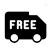##### Free Delivery